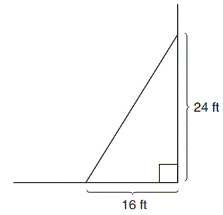Chapter 8.1, Problem 23EElementary Geometry For College St...

7th Edition
Alexander + 2 others
ISBN: 9781337614085

Solutions

Chapter
SectionElementary Geometry For College St...

7th Edition
Alexander + 2 others
ISBN: 9781337614085
Textbook Problem

A triangular corner of a store has been roped off to be used as an area for displaying Christmas ornaments. Find the area of the display section.To determine

To find:

The area of the display section

Explanation

Finding the area of the figure is followed by identifying the figure and find area with the suitable geometry formulae.

Calculation:

Given,

Lengths of the two legs a=16ft and b=24ft

Area of the right triangular corner A=12ab unit2

=12(16

Still sussing out bartleby?

Check out a sample textbook solution.

See a sample solution

The Solution to Your Study Problems

Bartleby provides explanations to thousands of textbook problems written by our experts, many with advanced degrees!

Get Started

If f and g are increasing on an interval I, then f g is increasing on I.

Single Variable Calculus: Early Transcendentals, Volume I

Convert the expressions in Exercises 31-36 to positive exponent form. 45y3/4

Finite Mathematics and Applied Calculus (MindTap Course List)

In Exercises 2336, find the domain of the function. 28. f(x)=x5

Applied Calculus for the Managerial, Life, and Social Sciences: A Brief Approach

Differentiate the functions in Problems 3-20. 16.

Mathematical Applications for the Management, Life, and Social Sciences

Simplify each power of i. i33

Trigonometry (MindTap Course List)

In Exercises 15-28, construct a truth table for the compound proposition. (pq)(qp)

Finite Mathematics for the Managerial, Life, and Social Sciences

The slope of the tangent line to r = cos θ at is:

Study Guide for Stewart's Single Variable Calculus: Early Transcendentals, 8th

Given the Taylor Series , a Taylor series for ex/2 is:

Study Guide for Stewart's Multivariable Calculus, 8th